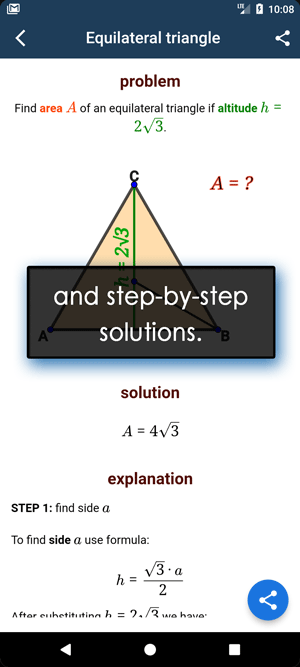Math Calculators, Lessons and Formulas

It is time to solve your math problem

mathportal.org

# Percentage calculator

This online calculator solves the four basic types of percent problems and percentage increase/decrease problem. The calculator will generate a step-by-step explanation for each type of percentage problem.

Percentage Calculator
Four types of percentage problems + steps.
show help ↓↓ examples ↓↓working...
examples
example 1:ex 1:
Calculate 45% of 120.
example 2:ex 2:
The number 18 is what percentage of 90?
example 3:ex 3:
The number 150 is 75% of what number?
example 4:ex 4:
What is the percentage increase from to 50 to 75?

This calculator solves four types of percentage problems.

Type 1: What is 15% of 20?

This can be solved in two steps:

Step 1: Write 15% as a decimal number: 15% = 0.15

Step 2: Multiply 0.15 and 20 to get the answer: 0.15 * 20 = 3

Type 2: The number 25 is what percentage of 80?

This can be solved in two steps:

Step 1: Solve the equation: 80 * x = 25. The solution is x = 0.3125

Step 2: Multiply 0.3125 by 100% to get the answer: 0.3125 * 100% = 31.25 %

Type 3: The number 32 is 8% of what number?

As usual, this problem requires to steps:

Step 1: Write 8% as a decimal number: 8% = 0.08

Step 2: Divide 32 and 0.08: 32 ÷ 0.08 = 400

Type 4: percentage increase: What is the percentage increase from 20 to 90?

In this type of problem we use formula

$$\text{Percentage increase} = \frac{\text{new amount} - \text{original amount}}{\text{original amount}} \cdot 100 \%$$

Using our numbers we have:

$$\text{Percentage increase} = \frac{90 - 20}{20} \cdot 100 \% = \frac{70}{20} \cdot 100 \% = 350 \%$$

Type 4: percentage decrease: What is the percentage decrease from 160 to 40?

Now we will use formula

$$\text{Percentage decrease} = \frac{\text{original amount} - \text{new amount}}{\text{original amount}} \cdot 100 \%$$

Using our numbers we have:

$$\text{Percentage decrease} = \frac{160 - 40}{160} \cdot 100 \% = \frac{120}{160} \cdot 100 \% = 75 \%$$
Search our database of more than 200 calculators